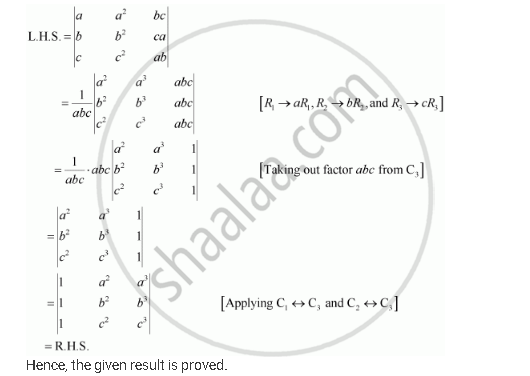Share

# Prove that the Determinant |(X,Sin Theta, Cos Theta),(-sin Theta, -x, 1),(Cos Theta, 1, X)| Is Independent Of θ.Is Independent Of θ. - CBSE (Commerce) Class 12 - Mathematics

ConceptDeterminants of Matrix of Order One and Two

#### Question

Prove that the determinant |(x,sin theta, cos theta),(-sin theta, -x, 1),(cos theta, 1, x)| is independent of θ.is independent of θ.

#### SolutionIs there an error in this question or solution?

#### Video TutorialsVIEW ALL 

Solution Prove that the Determinant |(X,Sin Theta, Cos Theta),(-sin Theta, -x, 1),(Cos Theta, 1, X)| Is Independent Of θ.Is Independent Of θ. Concept: Determinants of Matrix of Order One and Two.
S